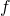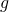# Function composition in C#

Function composition is about the essence of programming. Complex problems can be solved by decomposing them into many smaller problems that each can be worked out easily. Finally those small pieces have to be put together to form the overall solution. One way of combining these small pieces is function composition.

Also function composition is a great tool that makes the code more compact and reduces noise. Because of the concise syntax there are fewer possibilities to make mistakes like mixing up parameters e.g.

In this post I will show how function composition can be implemented in C# and how it is related to currying and partial application. Also I will discuss the pros and cons of function composition in C# and point out an alternative. All C# source code from this post can be downloaded here.

## Definition

Function composition is defined like this:. The composition operatorcombines the functionsandso that the output ofis pipelined intowhich creates an entirely new function. It is important that the input and output types match. A function g of type `(a -> b)` can be composed with a function f of type `(b -> c)` because the result of g is of type `b` which is the input type for f. The composed function therefore takes an argument of type `a` (which is the input type of g) and produces a `c` (which is the output type of f). In C# notation a function g of type `Func<T1, T2>` can be composed with a function f of type `Func<T2, T3>` to produce a function of type `Func<T1, T3>`.

In functional languages composition is usually a built-in higher-order function or operator. In Haskell the dot `.` is the composition operator. E.g. The functions `f` and `g` can be composed to create a new function `h` like this:

``````> let h = f . g
``````

The type signatures of the composition operators have to be in Haskell notation:
`(b -> c) -> (a -> b) -> a -> c`

or in C# notation:
`<Func<T2, T3>, Func<T1, T2>, Func<T1, T3>>`

## Implementation in C#

Since the composition operator is an infix operator we can imitate this in C# with extension methods. Here is the composition function in C#:

``````public static Func<T1, T3> Compose<T1, T2, T3>(this Func<T2, T3> f, Func<T1, T2> g)
{
return x => f(g(x));
}
``````

The following example shows how the composition function can be used:

``````var f = new Func<string, IEnumerable<string>>(s => s.Split(new[] {' '}));
var g = new Func<string, string>(s => s.ToUpper());

var h = f.Compose(g);

Assert.That(h("foo bar"), Is.EqualTo(new[]{ "FOO", "BAR" }));
``````

## Currying

In Haskell and F# functions are curried by default. That means that multiple input parameters can be applied to a function one by one. Each application of an argument returns a new curried function until the last argument is applied. By partially applying arguments it is possible to create functions that can easily be composed.

A function that takes a number, adds 1 and multiplies the result by 2 can be composed of the partially applied functions `(+)` and `(*)` like this in Haskell:

``````> let add1AndMultiplyBy2 = (* 2) . (+ 1)
``````

To be able to do this in C# nicely we need extension methods that convert uncurried functions to their curried form. E.g. a function of type `Func<T1, T2, T3>` in curried form will have the signature `Func<T1, Func<T2, T3>>`. When the first argument `T1` is applied the result is a function of type `Func<T2, T3>`. Next the argument `T2` can be applied and the result of that is a value of type `T3`.

Here are all the extension methods for converting functions with up to 16 input parameters. The example above can now be translated into C#:

``````// first we have to create curried versions of add and multiply
var add = new Func<int, int, int>((x, y) => x + y).Curry();
var multiply = new Func<int, int, int>((x, y) => x * y).Curry();

var add1AndMultiplBy2 = multiply(2).Compose(add(1));
``````

Note that also uncurried functions can be partially applied. But the syntax is way more clumsy:

``````var add1AndMultiplBy2 = new Func<int, int>(x => multiply(2, x))
.Compose(new Func<int, int>(x => add(x, 1)));
``````

## Function composition in practice

Let's examine a problem and it's solution in Haskell.

Problem:

Create a function that reverses all the words in a given string while the order of words has to stay the same.

Example:

``````> reverseWords "Foo bar" -- should return "ooF rab"
``````

Solution:

``````> let reverseWords = unwords . map reverse . words
``````

The input for `unwords` is the output of `map reverse` (where `map reverse` is a partially applied function). The input for `map reverse` again is the output of `words`. (All of these functions are defined in the standard Haskell Prelude module).

In order to translate this to C# we first need to define the four functions: Words, Unwords, Map and Reverse.

``````static readonly Func<string, IEnumerable<string>> Words =
s => s.Split(new[] { ' ' }, StringSplitOptions.RemoveEmptyEntries);

static readonly Func<Func<string, string>, IEnumerable<string>, IEnumerable<string>> Map =
(f, list) => list.Select(f);

static readonly Func<string, string> Reverse =
s => new String(s.Reverse().ToArray());

static readonly Func<IEnumerable<string>, string> Unwords =
list => String.Join(" ", list);
``````

Now we can compose these functions and check if the created function processes the input string correctly:

``````var reverseWords = Unwords
.Compose(Map.Curry()(Reverse))
.Compose(Words);

Assert.That(reverseWords("Foo bar"), Is.EqualTo("ooF rab"));
``````

## Evaluation of function composition in C#

The C# version with function composition looks quite good.

• The code is mostly declarative and therefore easy to understand
• No types have to be specified because type inference works well
• Function arguments don't have to be mentioned explicitly (which is also called pointfree style)

Still there are some drawbacks:

Readability
Compared to Haskell or F# readability is not quite as good. The code is not as concise and compact as it theoretically could be because of several reasons:

• The composition function has to have a name and cannot simply be a symbol (e.g. an overloaded operator)
• Parameters have to be enclosed in parentheses
• Currying has to be done explicitly

(Note that `Map` could have been defined in curried form easily. But this isn't always possible as many functions come from other libraries that cannot be changed.)

Order of application
With function composition as it is defined in mathematics and in Haskell and as it is shown here the order of application is from right to left. But as we read the code from left to right this can be confusing.

Off course the solution to this is easy. We could implement a "forward composition" function as exists in F# with the `(>>)` operator.

Usability
Functions have to be defined as (or assigned to) `Func<...>` delegates as properties, fields or local variables in order to apply composition. This is not always handy because "normal" methods have to be converted.

## Composition vs. pipelining

An alternative to function composition is pipelining. In F# pipelining can be done with the forward pipe operator `|>` which passes the result of the function on the left side to the function on the right side. Here is the example from above in F# using pipelining:

``````> "Foo bar" |> words |> Array.map reverse |> unwords;;
val it : string = "ooF rab"
``````

Pipelining in C# can be done with the help of extension methods. If we define the functions from the example above as extension methods we can compose the `reverseWords` function using the dot notation:

(Note that we don't need Map here because Select does the same thing.)

``````Func<string, string> reverseWords =
s => s.Words()
.Select(StringExtensions.Reverse)
.Unwords();
``````

The pros are:

• Simple infix operator
• Application from left to right
• It works without partial application
• It is more idiomatic to the C# language

Here is a brief comparison of the two approaches.

Function composition Pipelining
Simple operator (symbol) NO YES
Infix notation YES YES
Pointfree style YES NO
Application from left to right YES (with forward composition) YES
Works without partial application NO (not with multiple parameter functions) YES
Idiomatic NO (this is arguable) YES

## Conclusion

We've seen that function composition can easily be done in C#. Nevertheless it still doesn't feel as natural as it does in functional languages like F# or Haskell because the syntax is not as nice and clean.

If we want to do functional programming in C# a good alternative to function composition is pipelining with the use of extension methods.

Anyway if you want to get into functional programming and try to understand function composition, currying, partial application and higher-order functions it can be a good starting point and a good exercise to implement all of this in C# or in whatever language you are comfortable with.

All C# source code from this post can be downloaded here.